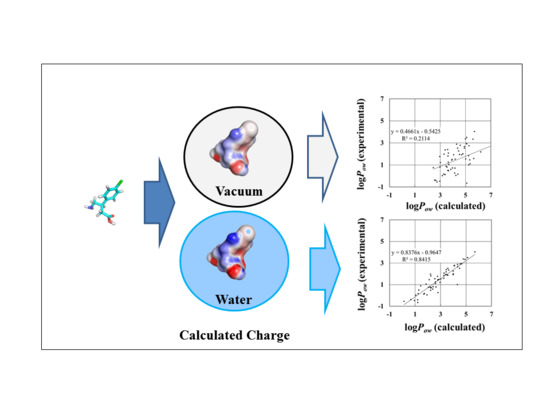Previous Article in Journal
Gold and Nickel Extended Thiophenic-TTF Bisdithiolene Complexes
Open AccessArticle

Effect of Atomic Charges on Octanol–Water Partition Coefficient Using Alchemical Free Energy Calculation

byKoji Ogata *,Makoto Hatakeyama andShinichiro Nakamura
RIKEN Innovation Center, Nakamura Laboratory, 2-1 Hirosawa, Wako, Saitama 351-0198, Japan
*
Author to whom correspondence should be addressed.
Molecules 2018, 23(2), 425; https://doi.org/10.3390/molecules23020425
Received: 19 January 2018 / Revised: 11 February 2018 / Accepted: 14 February 2018 / Published: 15 February 2018
(This article belongs to the Section Medicinal Chemistry)
The octanol–water partition coefficient (logPow) is an important index for measuring solubility, membrane permeability, and bioavailability in the drug discovery field. In this paper, the logPow values of 58 compounds were predicted by alchemical free energy calculation using molecular dynamics simulation. In free energy calculations, the atomic charges of the compounds are always fixed. However, they must be recalculated for each solvent. Therefore, three different sets of atomic charges were tested using quantum chemical calculations, taking into account vacuum, octanol, and water environments. The calculated atomic charges in the different environments do not necessarily influence the correlation between calculated and experimentally measured ∆Gwater values. The largest correlation coefficient values of the solvation free energy in water and octanol were 0.93 and 0.90, respectively. On the other hand, the correlation coefficient of logPow values calculated from free energies, the largest of which was 0.92, was sensitive to the combination of the solvation free energies calculated from the calculated atomic charges. These results reveal that the solvent assumed in the atomic charge calculation is an important factor determining the accuracy of predicted logPow values. View Full-Text
Show FiguresGraphical abstract

MDPI and ACS Style

Ogata, K.; Hatakeyama, M.; Nakamura, S. Effect of Atomic Charges on Octanol–Water Partition Coefficient Using Alchemical Free Energy Calculation. Molecules 2018, 23, 425.

Show more citation formats Show less citations formats
Note that from the first issue of 2016, MDPI journals use article numbers instead of page numbers. See further details here.

1